#Python基础——while、字符串、列表及操作

0
0
01. 云栖社区>
2. 博客>
3. 正文

## Python基础——while、字符串、列表及操作

techlee 2018-05-26 17:18:00 浏览805

Python基础——day3

while和if的用法基本类似，区别在于，if条件成立，则执行一次；while条件成立则重复执行，直到条件不成立。

1.while循环的格式：
while条件：

``````# while循环格式演示
a = 1
while a <= 5:
print(a)
print("这是第%d次打印" % a)
a += 1
``````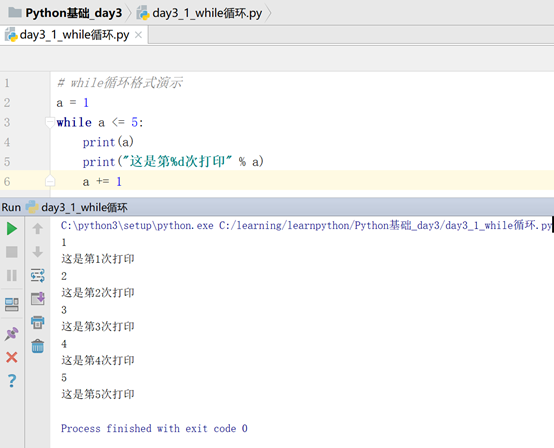image.png

2.while循环的应用

（1） 计算1-100的累计和

``````#需求:计算1-100的累计和
num = 1
num_sum = 0
while num <= 100:
num_sum = num_sum +num
num += 1
print(num_sum)
``````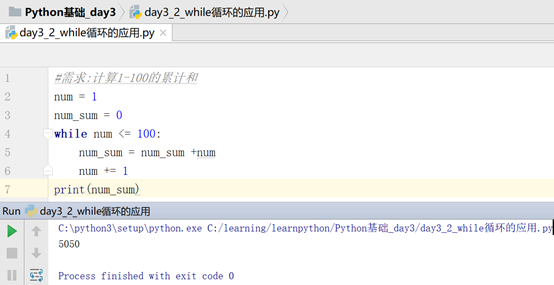image.png

（2） 计算1-100的偶数和

``````#需求：计算1-100的偶数和
#分析：偶数，即整数除以2，得到的结果没有余数，即余数为0
num = 1
num_sum = 0
while num <= 100:
if num % 2 == 0:
num_sum = num_sum + num
num += 1
print(num_sum)
``````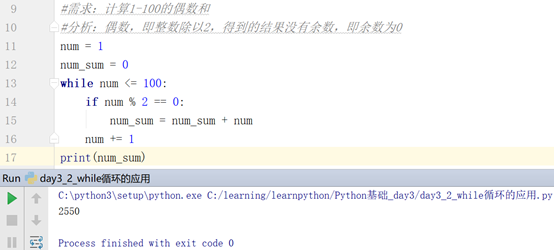image.png

3.while循环嵌套

While循环嵌套格式：
while条件1：

...
while条件2：

...
(1) while循环嵌套应用

``````*
**
***
****
``````

``````需求：打印如下格式图形
*
**
***
****

a = 1
while a <= 4:
b =1
while b <= a:
print("*", end="")  #因为print()打印时，有自动换行的特性，因此使用end=""来结束其换行
b += 1
a += 1
print()
``````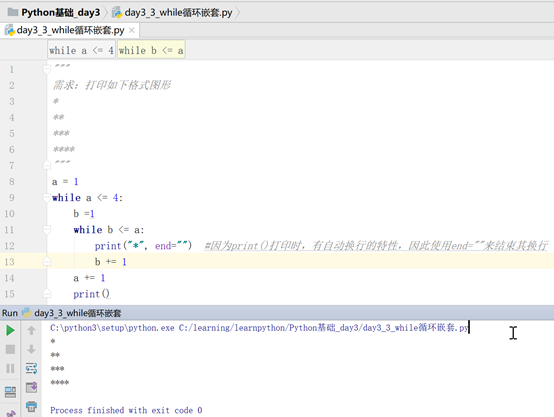image.png

（2） while循环制作九九乘法表

``````#需求，用while循环打印就就乘法表
a = 1
while a <= 9:
b = 1
while b <= a:
print("%d*%d=%d\t" % (b, a ,a*b),end="")
b += 1
a += 1
print()
``````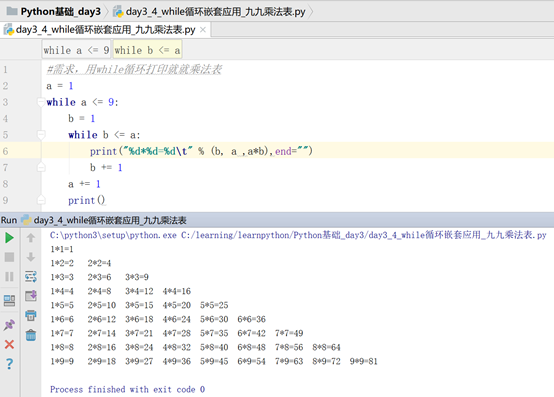image.png

（3） while循环打印倒九九乘法表

``````i = 9
while i>=1:
j = 1
while j<=i:
print("%d*%d=%d\t"%(j,i,j*i),end="")
j+=1
print("\n")
i-=1
``````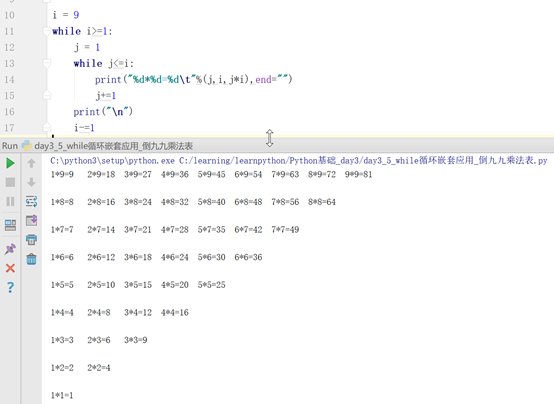image.png

break和continue 是两个只能在循环语句中使用到的关键词，有特殊作用。

1.（面试题）使用循环生成1，2，3，4，5，7，9，10数字

``````# #需求：使用循环生成1，2，3，4，5，7，9，10
my_num = 1
while my_num <= 10:
if my_num == 6 or my_num == 8:
my_num += 1                   #注意，使用continue的时候，要注意改变变量，否则构成死循环
continue                     #循环遇到continue之后，会直接跳到下一次循环的条件判断中
print( my_num )
my_num += 1
``````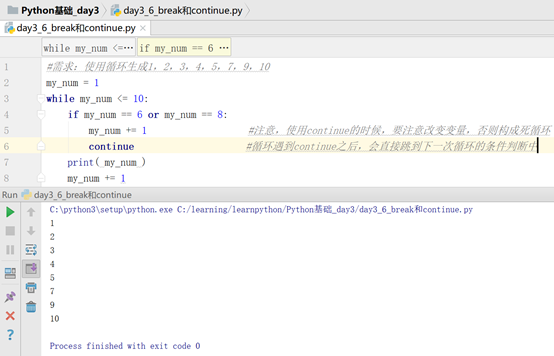image.png

2.修改上述需求，使用循环生成1-10数字，但是当数字是6时，结束循环

``````#修改需求：使用循环生成1-10数字，但是当数字是6时，结束循环
my_num = 1
while my_num <= 10:
if my_num == 6:
break        #循环中遇到break，会终止循环，执行循环以后的代码
print(my_num)
my_num += 1
``````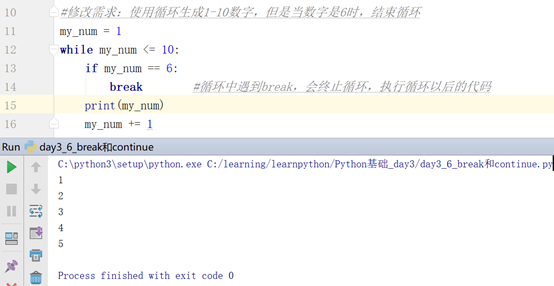image.png

1.字符串，就是文本，就是由多个字符组成的数据类型

Str = “hello,python”

``````# 字符串拼接
my_str1 = "hello"
my_str2 = "python"
my_str3 = my_str1 + "\t" + my_str2
print(my_str3)
``````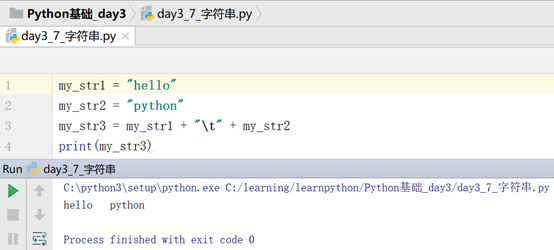image.png

``````#字符串和整数相乘
my_str1 = "hello,python \t"
print( my_str1*5 )
``````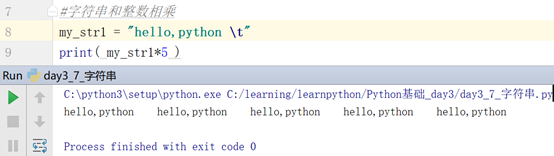image.png

（1） 使用单引号定义：

（2） 使用双引号定义：

（3） 使用三个单引号定义：

（4） 使用三个双引号定义：

``````# python中定义字符串的四种方法：
# 1.使用单引号定义
str1 = 'Hello,Python'
print(str1)
# 2.使用双引号定义
str2 = "Hello,World"
print(str2)
# 3.使用三个单引号定义
str3 = '''Hello,China'''
print(str3)
# 4.使用三个双引号定义
str4 = """Hello,Deutchland"""
print(str4)
``````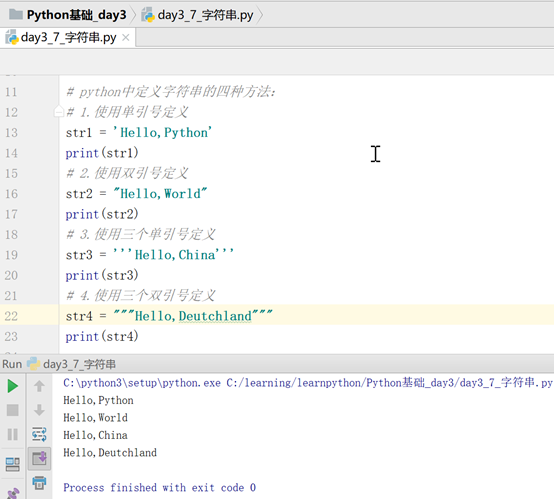image.png

2.字符串的相关操作

``````# 如果定义字符串的内容中含有英文单引号，则使用双引号进行定义
my_str1 = "I'm from China"
print(my_str1)
# 如果定义字符串的内容中含有英文双引号，则使用单引号进行定义
my_str2 = 'He said:"I am form England"'
print(my_str2)
``````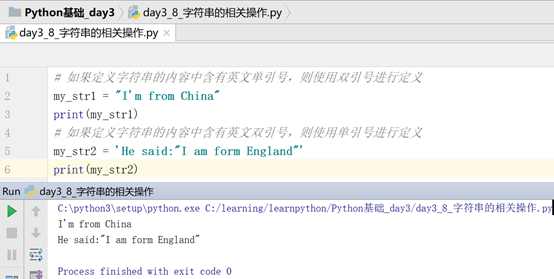image.png

1. 占位符：%
2. 转义字符：在字符串左边带着反斜杠的，\n表示换行，\t表示缩进一个tab
演示：
``````# 占位符
my_str1 = 5
print("小红上街买了%d个苹果" % my_str1)

#转义字符：\t  \n
my_str2 = "hello\npython"
print(my_str2)
my_str3 = "hello\tpython"
print(my_str3)
``````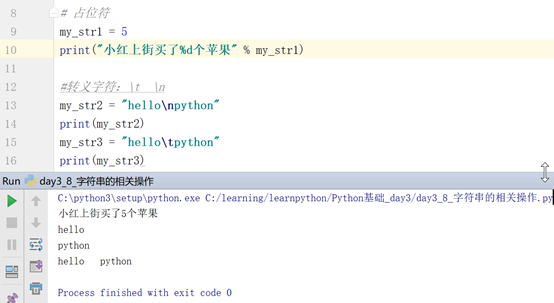image.png

\’和 \”，因为引号本身在字符串中表示字符的开始和结束，那么\’和\”表示，只是输出一个文本的引号。

``````# \' 和 \"
my_str1 = 'I\'m from China'
print(my_str1)
my_str2 = "He said :\" hello,python\""
print(my_str2)
``````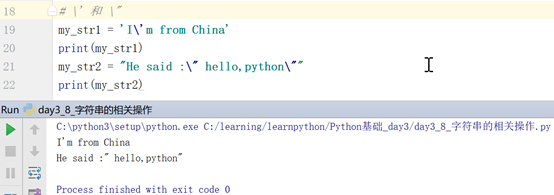image.png

Python中除了支持数字类型（整数，浮点数，布尔值）以外，还有非数字型（字符串、列表、元组、字典）

1.列表（list）：是一个由多个成员构成的数据类型

``````#需求保存一个班级同学的姓名：
al_class_student_name = ["小明", "小黑", "小白", "小红"]
print(al_class_student_name)
print(type(al_class_student_name))
``````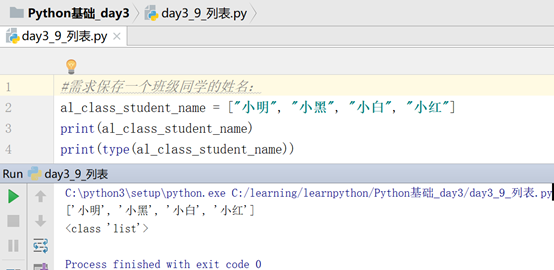image.png

1. 列表的下标
列表的下标指挥从0开始逐个递增
``````#列表的下标
my_list = ["a", "b", "c", "d", "e"]
print(my_list)
``````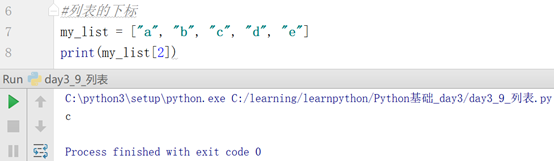image.png

``````#提取列表中倒数第一个数据
my_list =["a", "b", "c", "d", "e", "f"]
print(my_list[-1])
``````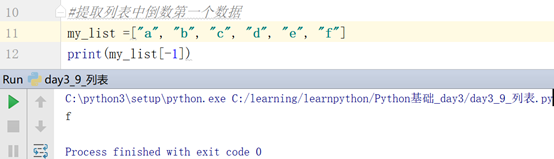image.png

1.for循环一般就是用来完成提取列表和字符串、元组的所有成员
for类似while，也是一种循环

``````class_student_list = ["小白", "小黑", "小灰", "小红", "小蓝"]
# 需求：希望把所有学生姓名全部输出出来
# 如果不使用循环来输出则如下：
print(class_student_list)
print(class_student_list)
print(class_student_list)
print(class_student_list)
print(class_student_list)
print()

#先使用while来完成：
a = 1
while a <= 4:
print(class_student_list[a])
a += 1
print()

#使用for循环实现：
for name in class_student_list:
print(name)
``````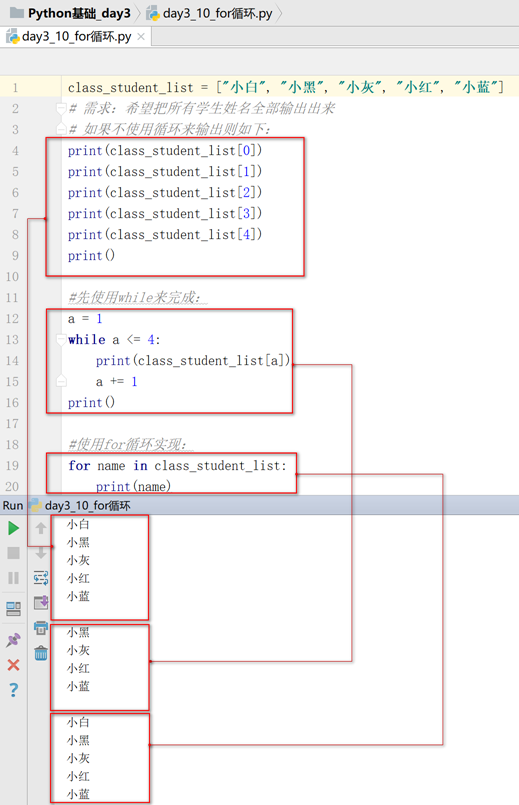image.png

2.for循环生成数字

``````# 需求：生成1-10之间的整数
# 先使用while循环实现：
a = 1
while a <= 10:
print(a, end="")  #使用end=""，让print()打印不换行
a += 1
print()

# 使用for循环实现：
# range(开始位置，结束位置) 可以生成指定范围的整数[不包含结束位置]
for i in range(1,11):
print(i, end="")
``````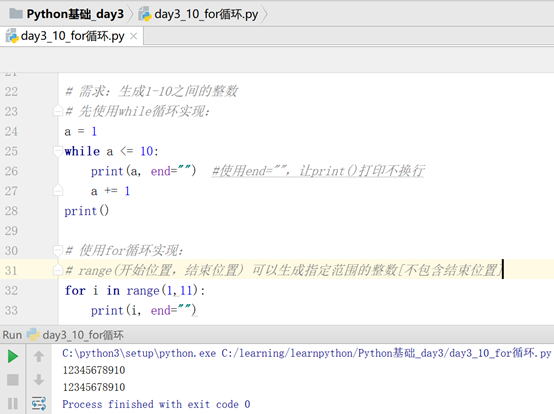image.png
1. for语句也可以有else语句
演示：
``````# for循环中的else用法：
my_list1 = ["小明", "小黑", "小白", "小红", "小蓝", "小灰", "小小黑"]
for name in my_list1:
if name == "小黑" :
print("找到你了，小黑")
break
else:
print("没找到你")    # for后面else语句中的代码会在执行所有循环后，才会执行，
# 遇到break,else就不会执行了
``````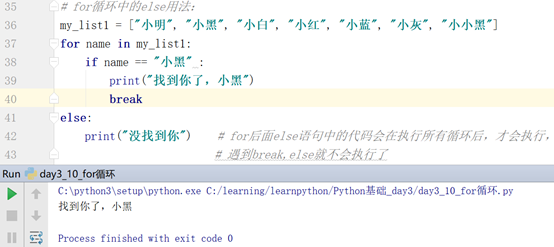image.png

（1） 列表也支持加法运算和乘法运算

``````#列表的加法运算，两个列表相加表示两个列表的拼接
list1 = [1,2,3]
list2 = ["a", "b", "c"]
list3 = list1 + list2
print(list3)

#列表的乘法运算，列表和整数相乘，表示按指定次数重复当前列表的成员
list4 = list1 * 4
print(list4)
``````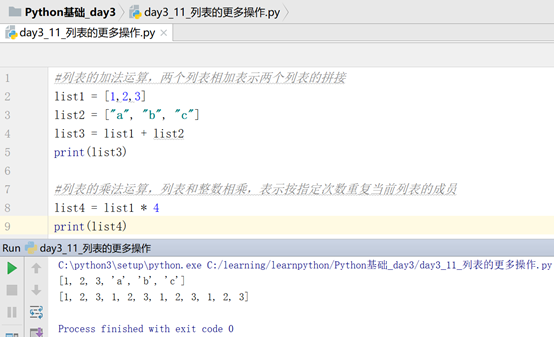image.png

（2） in 判断一个成员是否在列表中
in 是一个运算符，其结果只会是True和False。

``````# in 来判断成员是否在列表中
my_list = ["a", "b", "c", "d"]
print("a" in my_list)
``````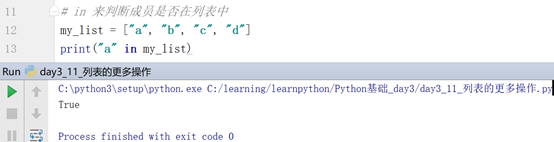image.png

True即表示该成员在列表中
（3） not in 判断一个成员是否不在列表中

``````# not in 判断一个成员是否不在这个列表中
my_list = ["a", "b", "c", "d"]
print("a" not in my_list)
``````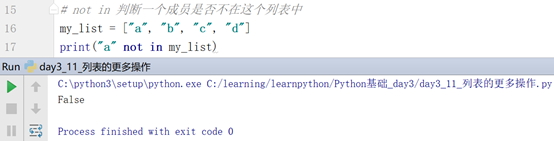image.png

``````# in 在开发中一般和if判断连用
my_list = ["a", "b", "c", "d"]
my_name = "a"
if my_name in my_list:
print("%s在列表中" % my_name)
else:
print("%s不在列表中" % my_name)
``````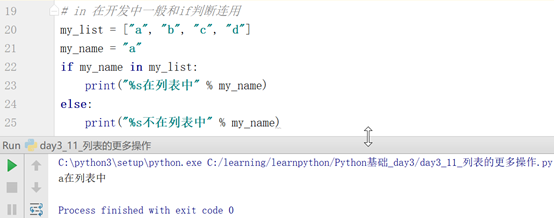image.png

（4） 列表的增、删、改、查

``````# 列表增加成员
# 1.在列表末尾追加成员
# 列表.append(数据)
my_list1 = ["a", "b", "c", "d"]
my_list1.append("e")
print(my_list1)

# 2.insert 指定下标左边位置插入数据
# 列表.insert(下标， 数据)
my_list2 = ["az", "bz", "cz", "dz"]
my_list2.insert(2, "fz")
print(my_list2)
# 在列表操作中，insert的下标如果不存在，则表示追加

# 3. extend 合并一个新的列表
my_list3 = ["ax", "bx", "cx", "dx"]
my_list4 = ["ex", "fx", "gx"]
my_list3.extend(my_list4)
print(my_list3)

# 列表删除成员操作
# 1.使用del通过指定下标可以删除成员
my_list5 = ["aa", "bb", "cc", "dd"]
del my_list5[-1]
print(my_list5)

# 2.使用remove删除成员
# 列表.remove(要删除的数据)
# 使用remove删除数据时，如果数据不存在会报错
my_list6 = ["aaa", "bbb", "ccc"]
my_list6.remove("aaa")
print(my_list6)

# 3.使用pop删除最后一个成员，也可以删除指定下标对应的数据
# 设置的下标必须存在，否则报错
my_list7 = ["aaaa","bbbb", "cccc", "dddd"]
my_list7.pop(-1)
print(my_list7)

# 4.清空列表
# 列表.clear()
my_list8 = ["q", "w", "e", "r"]
my_list8.clear()
print(my_list8)

# 列表修改成员的值
# 直接通过下标进行值的修改，如果下标不存在，则直接报错
my_list9 = ["t", "y", "u", "i"]
my_list9[-1] = "o"
print(my_list9)

# 列表查找成员
# 1.直接通过下标index查找成员，如果下标不存在，则报错
my_list10 = ["1", "2", "3", "4"]
print(my_list10)

# 2.通过值来查找成员下标，如果不存在，则报错
my_list11 = ["11", "22", "33", "44"]
res1 = my_list11.index("11")
print(res1)

# 3.在指定下标范围内查找数据，找不到则报错
my_list12 = ["z", "x", "c", "v"]
res2 = my_list12.index("x", 0, 2)   #查找的时候不包含结束位置
print(res2)

# 4.count 统计成员在列表中出现的次数，如果没有，则为0次
my_list13 = ["a", "s", "d", "a", "a", "f"]
res3 = my_list13.count("a")
print(res3)

# 5.len 统计列表成员的个数
my_list14 = ["qq", "ww", "ee", "rr"]
res4 = len(my_list14)
print(res4)

# 列表排序
# 让列表中的成员进行升序排序
my_list15 = [8, 2, 6, 7, 3, 1]
my_list15.sort()
print(my_list15)

# 可以通过key=str，来设置排序的时候，全部当成字符串来进行排序
my_list16 = ["a", 2, "f", 8, "s", "p", 3]
my_list16.sort(key=str)
print(my_list16)

# 可以通过key=len，来设置排序的时候，通过字符串长短来排序
# sort()默认让列表成员进行升序排列，还可以设置降序
my_list17 = [6, 4, 8, 0, 3, 5]
my_list17.sort(reverse=True)  #让列表中的成员降序排序
print(my_list17)

# reverse()可以将列表中成员进行逆序排列
my_list18 = ["小明", "小白", "小兰", "小黑"]
my_list18.reverse()
print(my_list18)
``````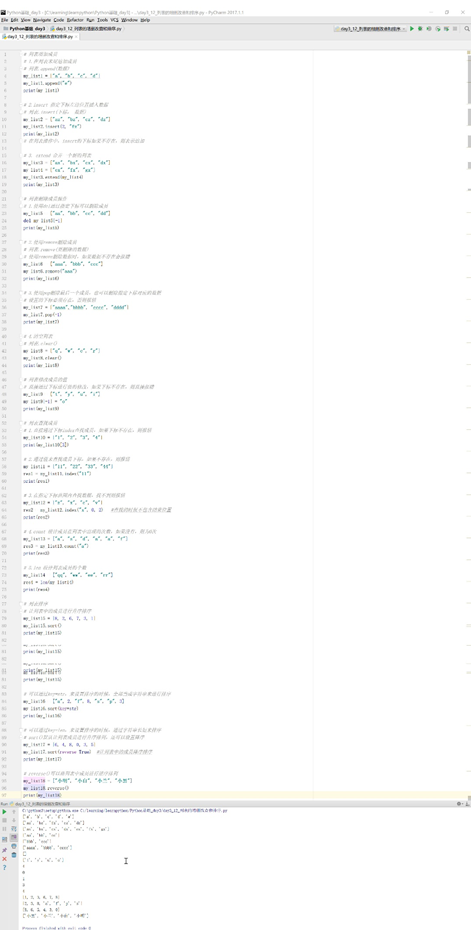image.png

techlee
+ 关注